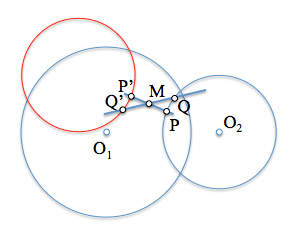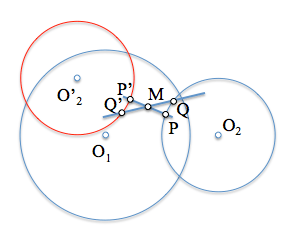# Determinación de un segmento conocido su punto medio [Solución]Al plantear un problema de geometría métrica podemos abordar su resolución con diferentes estrategias. to illustrate one of these methods solve we determine a segment is called the midpoint along with additional restrictions.

En particular analizaremos el caso en el que los extremos del segmento se encuentran situados sobre dos circunferencias coplanarias de radio arbitrario.

The problem statement is, therefore:

Determine the segments that are supported on two circles and having at point M as the midpoint.

The circles can have any radius and position, depending on the relative position of the crossing point M we find many different solutions to the problem.The method used in this case will be based on the analysis of loci to identify the points that meet the constraints of, being among them those that meet all.

Suppose the point P belongs to one of the solutions. This point we have placed on the circle with center O2. If you point out a solution, its symmetrical P’ to the midpoint M should be on the other circumference, and M is the midpoint.If we do this with another point, for example, the Q, Q end’ again will be symmetrical with respect to M Q. If the solution, would be on the other circumference. By repeating the operation with the infinite points of the circle with center O2, their symmetrical be found by determining a circumference previous symmetrical symmetry center point M.We can therefore determine the locus of all symmetric, to be found in the circle of equal radius centered, O2′, O2 will be symmetric.The points I1 and I2 of the circumference of intersection that are symmetrical with the other circumference of the center O1 of which must be located the ends of the segment determine the two possible solutions of the problem.The problem will take up to two solutions in this case, can not have any if the circles do not intersect.